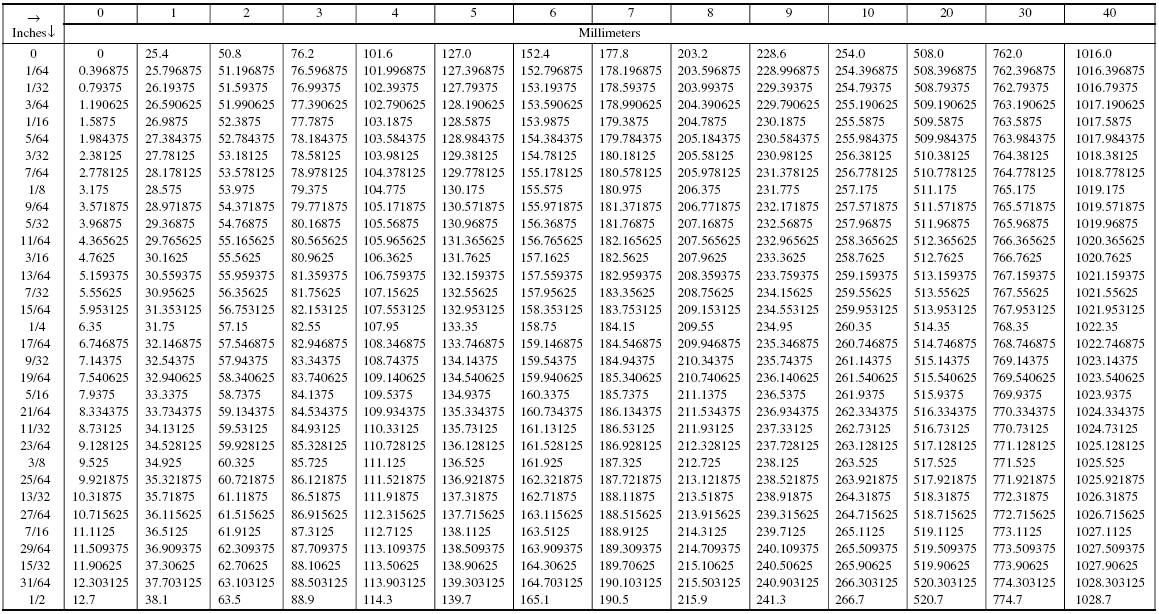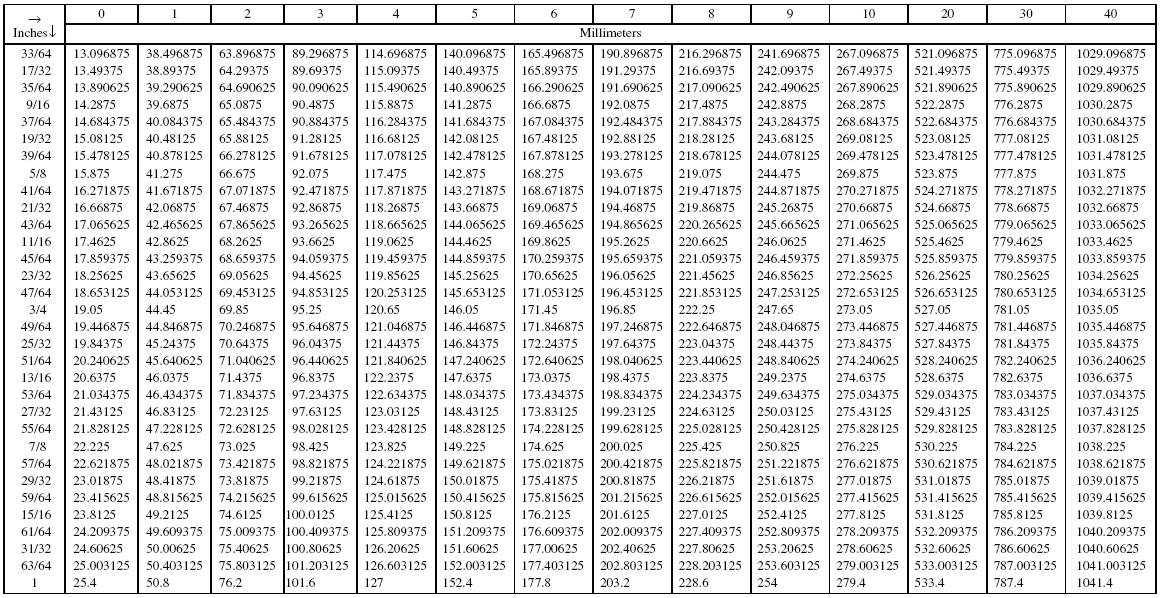Fractional Inches to Millimeters Conversion for 0 to 41 Inches in 1⁄64-Inch IncrementsBased on 1 inch = 25.4 millimeters, exactly.
All values in this table are exact.

Example: Convert 21 2364 inches to millimeters.

Solution:

From the first page of this table, find 20 inches = 508.0 millimeters

and add to that 1 2364 inches = 34.528125 millimeters
found at the intersection of the 1- inch column and the row containing 2364 inch .

Thus, 21 2364 inches = 508.0 + 34.528125 = 542.528125 mm, exactly.

Recherche personnalisée